SAS Congruency Criteria

Congruence of Triangles
Concept wise

To prove two triangles congruent,

We use SAS Criteria – Side Angle Side

In SAS Congruency Criteria,

• 2 sides of both the triangles are equal
• 1 angle between these side of both the triangles are equal.

For example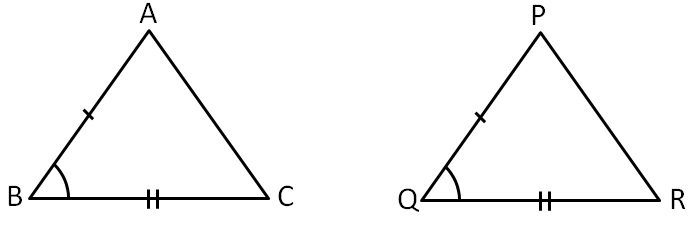Here,

2 sides and the angle between  them are equal.

Let’s do some examples

### Are these triangles congruent?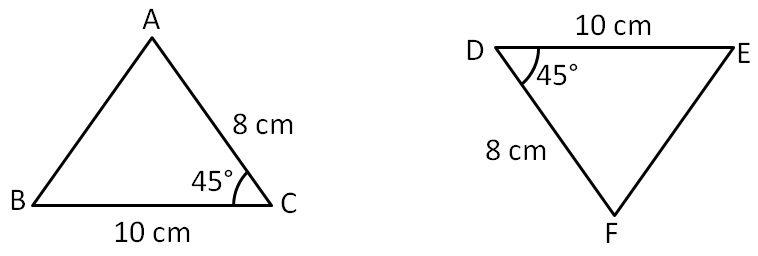In ∆ABC and ∆FED,

BC = ED      (Both are 10 cm )

∠C = ∠D      (Both are 45 °)

AC = FD      ( Both are 8 cm )

∴ ∆ABC ≅ ∆FED     (SAS Congruence Rule)

Here,

C D

A F

B E

### Are these triangles congruent?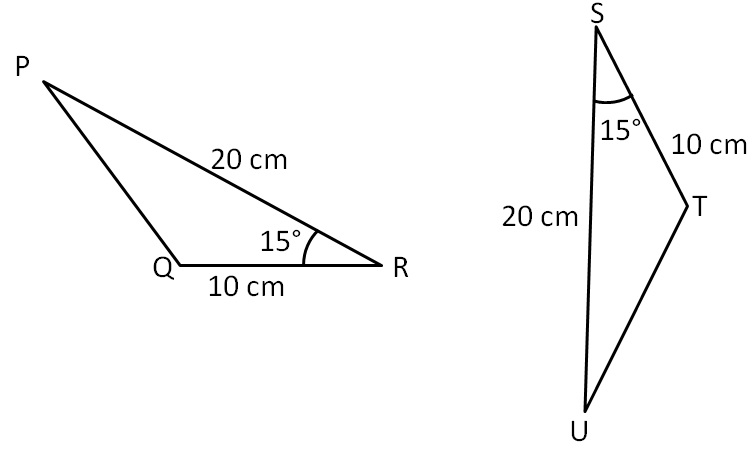In ∆PQR and ∆UTS,

PR = US     (Both are 20 cm )

∠R = ∠S     (Both are 15 °)

QR = TS     (Both are 10 cm )

∴ ∆PQR ≅ ∆UTS  (SAS Congruence Rule)

Here,

R S

P U

Q T

### Are these triangles congruent?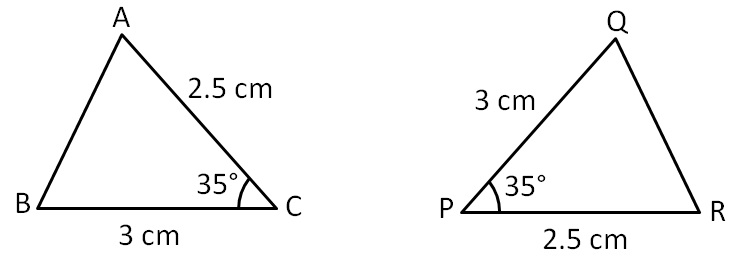In ∆ABC and ∆RQP,

AC = RP  ( Both are 2.5 cm )

∠C = ∠P  ( Both are 35 °)

BC = QP  ( Both are 3 cm )

∴ ∆ABC ≅ ∆RQP ( SAS Congruence Rule )

Here,

C P

A R

B Q

### Are these triangles congruent?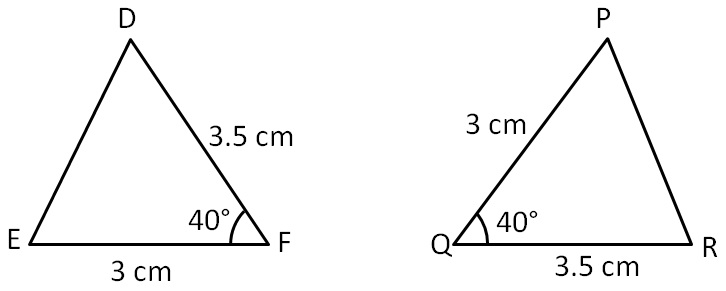In ∆DEF and ∆RPQ,

EF = PQ  (Both are 3 cm )

∠F = ∠Q  (Both are 40 °)

DF = RQ  (Both are 3.5 cm )

∴ ∆DEF ≅ ∆RPQ  (SAS Congruence Rule )

Here,

F Q

D R

E P

### Are these triangles congruent?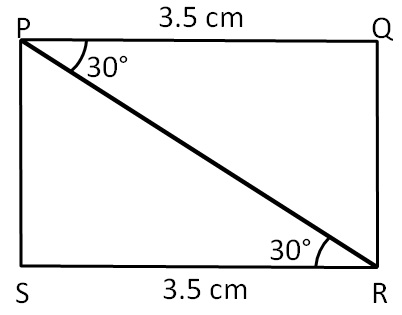In ∆PRS and ∆RPQ,

RS = PQ     ( Both are 3.5 cm )

∠R = ∠P     (Both are 30 °)

PR = RP    (Common )

∴ ∆PRS ≅ ∆RPQ  ( SAS Congruence Rule)

Here,

P R

S Q

R P

#### In the following diagram:-

In Fig, AB and CD bisect each other at O.

(i) State the three pairs of equal parts in two triangles AOC and BOD.

(ii) Which of the following statements are true?

(a) ∆AOC ≅ ∆DOB

(b) ∆AOC ≅ ∆BOD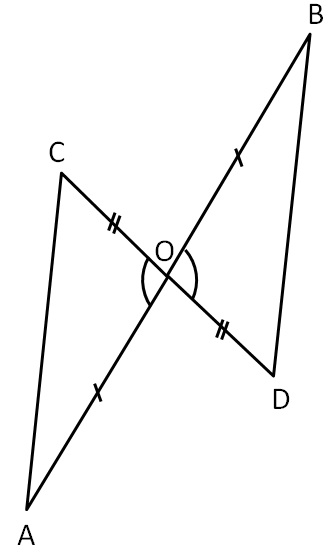In ∆AOC and ∆BOD,

OA = OB                      (Given )

∠AOC = ∠BOD          (Vertically opposite angles)

OC = OD                    ( Given)

∴ ∆AOC ≅ ∆BOD      ( SAS Congruence Rule )

Here,

A B

O O

C D

Learn in your speed, with individual attention - Teachoo Maths 1-on-1 Class